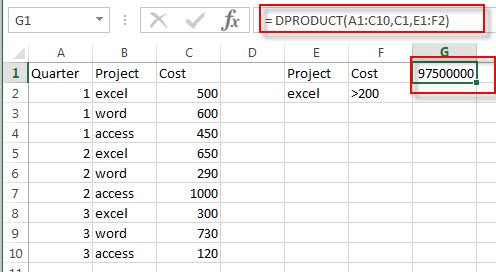# Excel DPRODUCT Function

This post will guide you how to use Excel DPRODUCT function with syntax and examples in Microsoft excel.

### Description

The Excel DPRODUCT returns the product of values from a set of records that match criteria that you specify.

The DPRODUCT function is a build-in function in Microsoft Excel and it is categorized as a Database Function.

The DPRODUCT function is available in Excel 2016, Excel 2013, Excel 2010, Excel 2007, Excel 2003, Excel XP, Excel 2000, Excel 2011 for Mac.

### Syntax

The syntax of the DPRODUCT function is as below:

`=DPRODUCT(database, field, criteria)`

Where the DPRODUCT function arguments are:

• Database -This is a required argument. The range of cells that containing the database.
• Field – This is a required argument. The column with database that you want the minimum of.
• Criteria – The range of cells that contains the conditions that you specify.

### Excel DPRODUCT Function Examples

The below examples will show you how to use Excel DPRODUCT Function to calculate the product of value from a column of a list or database that matches a given criteria.

#1 to get the product of the Cost from excel project with a cost is greater than 200, using the following formula:

`= DPRODUCT(A1:C10,C1,E1:F2)`Sidebar